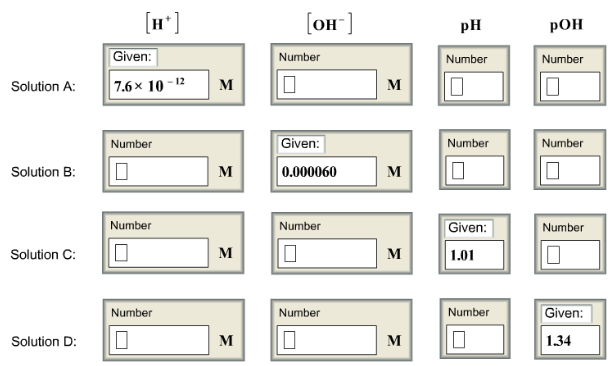# Problem: Complete this table of values for four aqueous solutions at 25  °C.

###### FREE Expert Solution

The pH and pOH of a compound determine its acidity and basicity.

• pH

▪ pH stands for the negative logarithmic function of hydronium ion concentration
can be calculated using the following equation:

Take note that:         [H+] = [H3O+]

• pOH

▪ pOH stands for the negative logarithmic function of hydroxide concentration
can be calculated using the following equation:

The relationship between pH and pOH is connected by the following equation:

$\overline{){\mathbf{pH}}{\mathbf{+}}{\mathbf{pOH}}{\mathbf{=}}{\mathbf{14}}}$

On the other hand, if the pH and pOH are given:

The H+ or H3O+ concentration can be calculated using the following equation:

$\overline{)\left[{\mathbf{H}}^{\mathbf{+}}\right]{\mathbf{=}}{\mathbf{10}}^{\mathbf{-}\mathbf{pH}}}$

The OH- concentration can be calculated using the following equation:

$\overline{)\left[{\mathbf{OH}}^{\mathbf{-}}\right]{\mathbf{=}}{{\mathbf{10}}}^{\mathbf{-}\mathbf{pOH}}}$

The relationship between [H+] and [OH-] is connected by the following equation:

$\overline{){{\mathbf{K}}}_{{\mathbf{w}}}{\mathbf{=}}\left[{\mathbf{H}}^{\mathbf{+}}\right]\left[{\mathbf{OH}}^{\mathbf{-}}\right]}$

Kw = autoionization constant of water

Kw = 1.0x10-14 at T = 25°C

81% (378 ratings)###### Problem Details

Complete this table of values for four aqueous solutions at 25  °C.